## UPDATE Statment

`UPDATE` statement is a SQL DML statement used to change and update the data in tables. We will explain the UPDATE statement with sample applications. Let’s take a look at how this expression is used.

``````UPDATE table_name SET = column1 = new_value, column2 = new_value WHERE condition
``````

Now let’s write our codes, for this, let’s see our table first.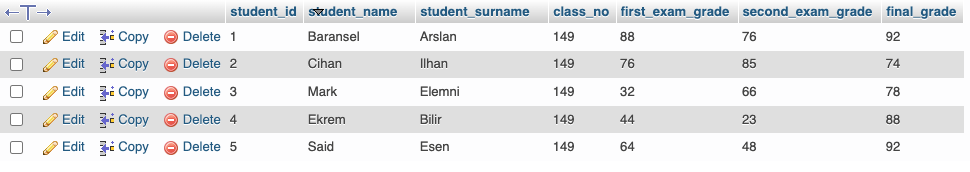For example, let’s add 10 points to the second exam grade of the student whose name is Baransel;

``````UPDATE students SET second_exam_grade = second_exam_grade + 10 WHERE student_name = 'Baransel'
``````

Now let’s look at our table;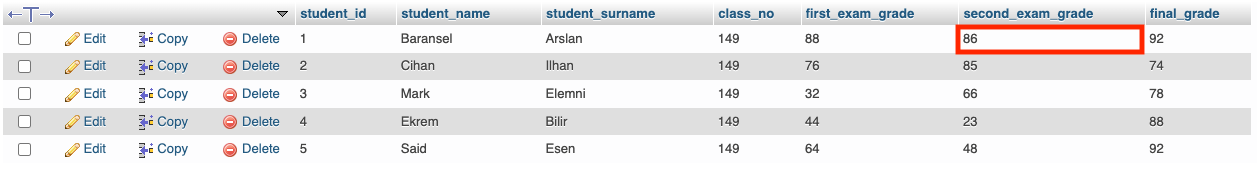Let’s do another example, this time, let’s add 10 points to both the first exam grade and the final grade of all students whose first exam grade is less than 50.

``````UPDATE students SET first_exam_grade = first_exam_grade + 10, final_grade = final_grade + 10 WHERE first_exam_grade < 50
``````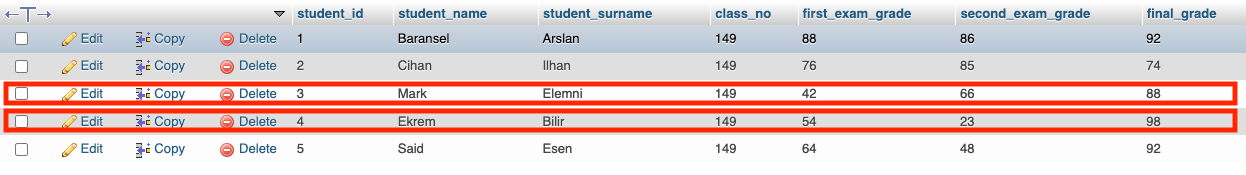## DELETE Statment

The `DELETE` statement is the SQL Data Manipulation Language statement used to delete data in a table. With the `DELETE` statement, the entire data in the table or the data that meets a certain condition is deleted.

``````DELETE FROM table_name WHERE condition
``````

For example, let’s delete students whose surnnames does not start with the letter “E” from the table;

``````DELETE FROM students WHERE student_surname NOT LIKE 'E%'
``````

Thus, we deleted 3 students from the table. Let’s take a look at the final version of our table.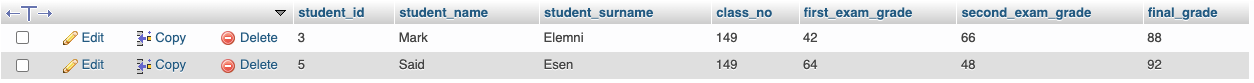Now let’s delete all the data in our table.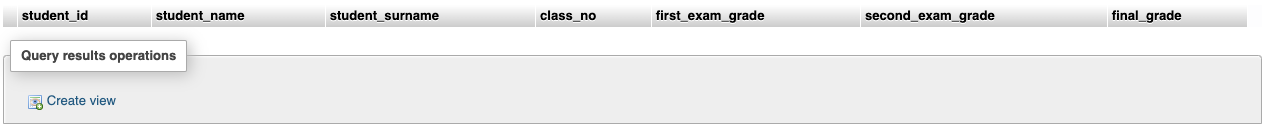``````DELETE FROM students
``````

As you can see we have deleted all the data inside our table. The point to note here is that we can delete the data in the table with the `DELETE` statement, but not the tables. If you want to delete the table, not the data in the table, you can refer to the `DROP` statement in the SQL DDL Commands.